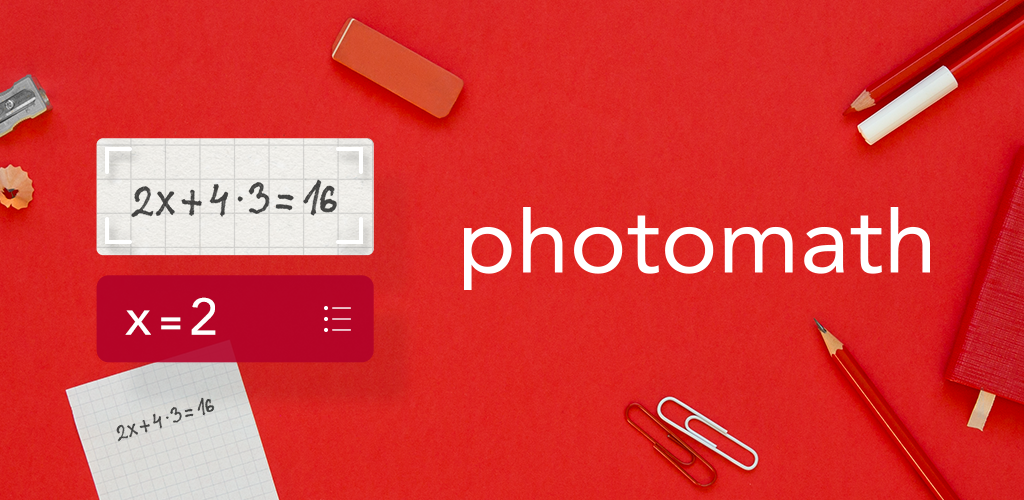# Photomath for smartphonesLearn how to solve math problems, check homework assignments and study for upcoming exams and ACTs/SATs with the world’s most used math learning resource. Photomath is FREE and works without wi-fi.

### How it works

Instantly scan printed text AND handwritten math problems using your device’s camera or type and edit equations with our scientific calculator. Photomath breaks down every math problem into simple, easy-to-understand steps so you can really understand core concepts and can answer things confidently.

### Features

• Scan textbook (print) AND handwritten problems
• Scientific calculator
• Step-by-step explanations for every solution
• Multiple solving methods
• No internet connection required to use
• 30+ languages supported
• Interactive graphs

### Maths Topics

• Basic Math/Pre-Algebra: arithmetic, integers, fractions, decimal numbers, powers, roots, factors
• Algebra: linear equations/inequalities, quadratic equations, systems of equations, logarithms, functions, matrices, graphing, polynomials
• Trigonometry/Precalculus: identities, conic sections, vectors, matrices, complex numbers, sequences and series, logarithmic functions
• Calculus: limits, derivatives, integrals, curve sketching
• Statistics: combinations, factorials#### Michael Osei-Owusu

Michael Osei-Owusu is a Ghanaian EduTech blogger and a teacher.